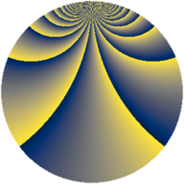# Properties

 Label 1110.2.bzLevel $1110$ Weight $2$ Character orbit 1110.bz Rep. character $\chi_{1110}(131,\cdot)$ Character field $\Q(\zeta_{36})$ Dimension $624$ Sturm bound $456$

# Related objects

## Defining parameters

 Level: $$N$$ $$=$$ $$1110 = 2 \cdot 3 \cdot 5 \cdot 37$$ Weight: $$k$$ $$=$$ $$2$$ Character orbit: $$[\chi]$$ $$=$$ 1110.bz (of order $$36$$ and degree $$12$$) Character conductor: $$\operatorname{cond}(\chi)$$ $$=$$ $$111$$ Character field: $$\Q(\zeta_{36})$$ Sturm bound: $$456$$

## Dimensions

The following table gives the dimensions of various subspaces of $$M_{2}(1110, [\chi])$$.

Total New Old
Modular forms 2832 624 2208
Cusp forms 2640 624 2016
Eisenstein series 192 0 192

## Trace form

 $$624q + O(q^{10})$$ $$624q + 72q^{21} - 48q^{28} + 24q^{31} + 120q^{34} + 48q^{37} + 96q^{43} + 48q^{45} + 96q^{49} - 72q^{54} + 72q^{57} - 48q^{61} + 72q^{63} + 168q^{69} + 96q^{78} + 48q^{79} + 96q^{81} + 48q^{82} + 144q^{87} + 192q^{91} - 144q^{93} - 144q^{94} - 48q^{97} - 72q^{99} + O(q^{100})$$

## Decomposition of $$S_{2}^{\mathrm{new}}(1110, [\chi])$$ into newform subspaces

The newforms in this space have not yet been added to the LMFDB.

## Decomposition of $$S_{2}^{\mathrm{old}}(1110, [\chi])$$ into lower level spaces

$$S_{2}^{\mathrm{old}}(1110, [\chi]) \cong$$ $$S_{2}^{\mathrm{new}}(111, [\chi])$$$$^{\oplus 4}$$$$\oplus$$$$S_{2}^{\mathrm{new}}(222, [\chi])$$$$^{\oplus 2}$$$$\oplus$$$$S_{2}^{\mathrm{new}}(555, [\chi])$$$$^{\oplus 2}$$